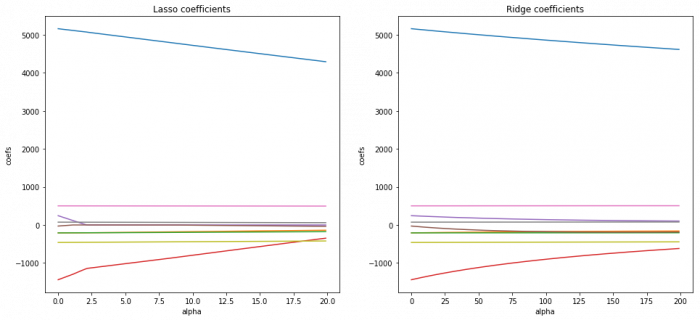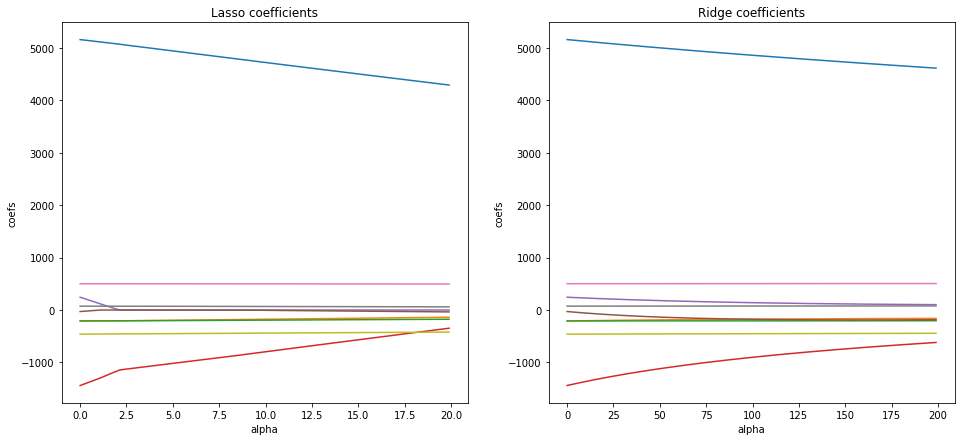# Ridge and Lasso in PythonFor many machine learning problems with a large number of features or a low number of observations, a linear model tends to overfit and variable selection is tricky. Models that use shrinkage such as Lasso and Ridge can improve the prediction accuracy as they reduce the estimation variance while providing an interpretable final model.In this tutorial, we will examine Ridge and Lasso regressions, compare it to the classical linear regression and apply it to a dataset in Python. Ridge and Lasso build on the linear model, but their fundamental peculiarity is regularization. The goal of these methods is to improve the loss function so that it depends not only on the sum of the squared differences but also on the regression coefficients.

One of the main problems in the construction of such models is the correct selection of the regularization parameter. Сomparing to linear regression, Ridge and Lasso models are more resistant to outliers and the spread of data. Overall, their main purpose is to prevent overfitting.

The main difference between Ridge regression and Lasso is how they assign a penalty term to the coefficients. We will explore this with our example, so let's start.

We will work with the Diamonds dataset, which is freely available online: http://vincentarelbundock.github.io/Rdatasets/datasets.html. It contains the prices and other attributes of almost 54,000 diamonds. We will be predicting the price using the available attributes and compare the results for Ridge, Lasso, and OLS.

In :
```# Import libraries
import numpy as np
import pandas as pd
```
Out:

Unnamed: 0 carat cut color clarity depth table price x y z
0 1 0.23 Ideal E SI2 61.5 55.0 326 3.95 3.98 2.43
1 2 0.21 Premium E SI1 59.8 61.0 326 3.89 3.84 2.31
2 3 0.23 Good E VS1 56.9 65.0 327 4.05 4.07 2.31
3 4 0.29 Premium I VS2 62.4 58.0 334 4.20 4.23 2.63
4 5 0.31 Good J SI2 63.3 58.0 335 4.34 4.35 2.75
In :
```# Drop the index
diamonds = diamonds.drop(['Unnamed: 0'], axis=1)
```
Out:

carat cut color clarity depth table price x y z
0 0.23 Ideal E SI2 61.5 55.0 326 3.95 3.98 2.43
1 0.21 Premium E SI1 59.8 61.0 326 3.89 3.84 2.31
2 0.23 Good E VS1 56.9 65.0 327 4.05 4.07 2.31
3 0.29 Premium I VS2 62.4 58.0 334 4.20 4.23 2.63
4 0.31 Good J SI2 63.3 58.0 335 4.34 4.35 2.75
In :
```# Print unique values of text features
print(diamonds.cut.unique())
print(diamonds.clarity.unique())
print(diamonds.color.unique())
```

```['Ideal' 'Premium' 'Good' 'Very Good' 'Fair']
['SI2' 'SI1' 'VS1' 'VS2' 'VVS2' 'VVS1' 'I1' 'IF']
['E' 'I' 'J' 'H' 'F' 'G' 'D']
```

As you can see, there are a finite number of variables, so we can transform these categorical variables to numerical variables.

In :
```# Import label encoder
from sklearn.preprocessing import LabelEncoder
categorical_features = ['cut', 'color', 'clarity']
le = LabelEncoder()
# Convert the variables to numerical
for i in range(3):
new = le.fit_transform(diamonds[categorical_features[i]])
diamonds[categorical_features[i]] = new
```
Out:

carat cut color clarity depth table price x y z
0 0.23 2 1 3 61.5 55.0 326 3.95 3.98 2.43
1 0.21 3 1 2 59.8 61.0 326 3.89 3.84 2.31
2 0.23 1 1 4 56.9 65.0 327 4.05 4.07 2.31
3 0.29 3 5 5 62.4 58.0 334 4.20 4.23 2.63
4 0.31 1 6 3 63.3 58.0 335 4.34 4.35 2.75

Before building the models, let's first scale data. Lasso and Ridge put constraints on the size of the coefficients associated to each variable. But, this value depends on the magnitude of each variable and it is therefore necessary to center and reduce, or standardize, the variables.

In :
```# Import StandardScaler
from sklearn.preprocessing import StandardScaler
# Create features and target matrixes
X = diamonds[['carat', 'depth', 'table', 'x', 'y', 'z', 'clarity', 'cut', 'color']]
y = diamonds[['price']]
# Scale data
scaler = StandardScaler()
scaler.fit(X)
X = scaler.transform(X)
```

Now, we can basically build the Lasso and Ridge models. But for now, we will train it on the whole dataset and look at an R-squared score and on the model coefficients. Note, that we are not setting the alpha, it is defined as 1.

In :
```# Import linear models
from sklearn import linear_model
from sklearn.metrics import mean_squared_error
# Create lasso and ridge objects
lasso = linear_model.Lasso()
ridge = linear_model.Ridge()
# Fit the models
lasso.fit(X, y)
ridge.fit(X, y)
# Print scores, MSE, and coefficients
print("lasso score:", lasso.score(X, y))
print("ridge score:",ridge.score(X, y))
print("lasso MSE:", mean_squared_error(y, lasso.predict(X)))
print("ridge MSE:", mean_squared_error(y, ridge.predict(X)))
print("lasso coef:", lasso.coef_)
print("ridge coef:", ridge.coef_)
```

```lasso score: 0.8850606039595762
ridge score: 0.8850713120355513
lasso MSE: 1829298.919415987
ridge MSE: 1829128.4968064611
lasso coef: [ 5159.45245224  -217.84225841  -207.20956411 -1250.0126333
16.16031486    -0.           496.17780105    72.11296318
-451.28351376]
ridge coef: [[ 5.20114712e+03 -2.20844296e+02 -2.08496831e+02 -1.32579812e+03
5.36297456e+01 -1.67310953e+00  4.96434236e+02  7.26648505e+01
-4.53187286e+02]]
```

These two models give very similar results. So, we will split the data into training and test sets, build Ridge and Lasso, and choose the regularization parameter with the help of GridSearch. For that, we have to define the set of parameters for GridSearch. In this case, the models with the highest R-squared score will give us the best parameters.

In :
```# Make necessary imports, split data into training and test sets, and choose a set of parameters
from sklearn.model_selection import train_test_split
from sklearn.model_selection import GridSearchCV
import warnings
warnings.filterwarnings("ignore")

X_train, X_test, y_train, y_test = train_test_split(X, y, test_size=0.25, random_state=101)
parameters = {'alpha': np.concatenate((np.arange(0.1,2,0.1), np.arange(2, 5, 0.5), np.arange(5, 25, 1)))}

linear = linear_model.LinearRegression()
lasso = linear_model.Lasso()
ridge = linear_model.Ridge()
gridlasso = GridSearchCV(lasso, parameters, scoring ='r2')
gridridge = GridSearchCV(ridge, parameters, scoring ='r2')

# Fit models and print the best parameters, R-squared scores, MSE, and coefficients
gridlasso.fit(X_train, y_train)
gridridge.fit(X_train, y_train)
linear.fit(X_train, y_train)
print("ridge best parameters:", gridridge.best_params_)
print("lasso best parameters:", gridlasso.best_params_)
print("ridge score:", gridridge.score(X_test, y_test))
print("lasso score:", gridlasso.score(X_test, y_test))
print("linear score:", linear.score(X_test, y_test))
print("ridge MSE:", mean_squared_error(y_test, gridridge.predict(X_test)))
print("lasso MSE:", mean_squared_error(y_test, gridlasso.predict(X_test)))
print("linear MSE:", mean_squared_error(y_test, linear.predict(X_test)))
print("ridge best estimator coef:", gridridge.best_estimator_.coef_)
print("lasso best estimator coef:", gridlasso.best_estimator_.coef_)
print("linear coef:", linear.coef_)
```

```ridge best parameters: {'alpha': 24.0}
lasso best parameters: {'alpha': 1.5000000000000002}
ridge score: 0.885943268935384
lasso score: 0.8863841649966073
linear score: 0.8859249267960946
ridge MSE: 1812127.0709091045
lasso MSE: 1805122.1385342914
linear MSE: 1812418.4898094584
ridge best estimator coef: [[ 5077.4918518   -196.88661067  -208.02757232 -1267.11393653
208.75255168   -91.36220706   502.04325405    74.65191115
-457.7374841 ]]
lasso best estimator coef: [ 5093.28644126  -207.62814092  -206.99498254 -1234.01364376
67.46833024    -0.           501.11520439    73.73625478
-457.08145762]
linear coef: [[ 5155.92874335  -208.70209498  -208.16287626 -1439.0942139
243.82503796   -28.79983655   501.31962765    73.93030707
-459.94636759]]
```

Our score raises a little, but with these values of alpha, there is only a small difference. Let's build coefficient plots to see how the value of alpha influences the coefficients of both models.

In :
```# Import library for visualization
import matplotlib.pyplot as plt
coefsLasso = []
coefsRidge = []
# Build Ridge and Lasso for 200 values of alpha and write the coefficients into array
alphasLasso = np.arange (0, 20, 0.1)
alphasRidge = np.arange (0, 200, 1)
for i in range(200):
lasso = linear_model.Lasso(alpha=alphasLasso[i])
lasso.fit(X_train, y_train)
coefsLasso.append(lasso.coef_)
ridge = linear_model.Ridge(alpha=alphasRidge[i])
ridge.fit(X_train, y_train)
coefsRidge.append(ridge.coef_)

# Build Lasso and Ridge coefficient plots
plt.figure(figsize = (16,7))

plt.subplot(121)
plt.plot(alphasLasso, coefsLasso)
plt.title('Lasso coefficients')
plt.xlabel('alpha')
plt.ylabel('coefs')

plt.subplot(122)
plt.plot(alphasRidge, coefsRidge)
plt.title('Ridge coefficients')
plt.xlabel('alpha')
plt.ylabel('coefs')

plt.show()
```As a result, you can see that when we raise the alpha in Ridge regression, the magnitude of the coefficients decreases, but never attains zero. The same scenario in Lasso influences less on the large coefficients, but the small ones Lasso reduces to zeroes. Therefore Lasso can also be used to determine which features are important to us and keeps the features that may influence the target variable, while Ridge regression gives uniform penalties to all the features and in such way reduces the model complexity and prevents multicollinearity.